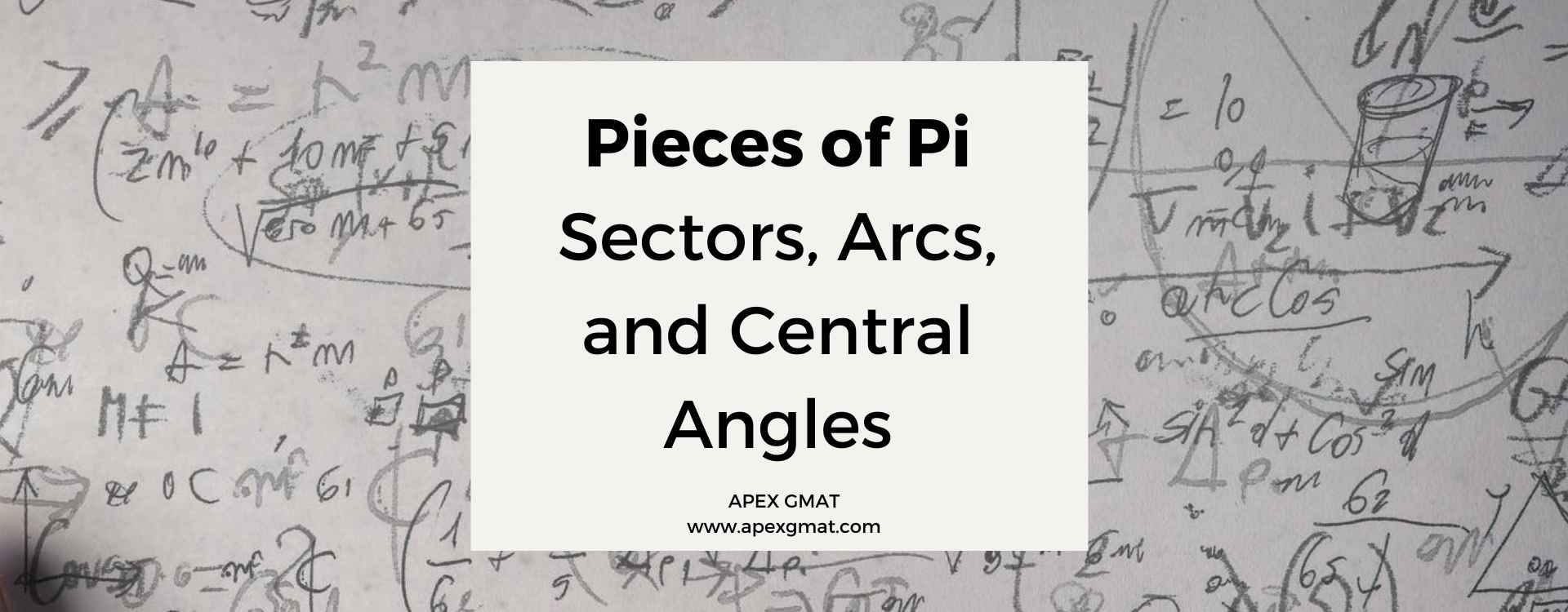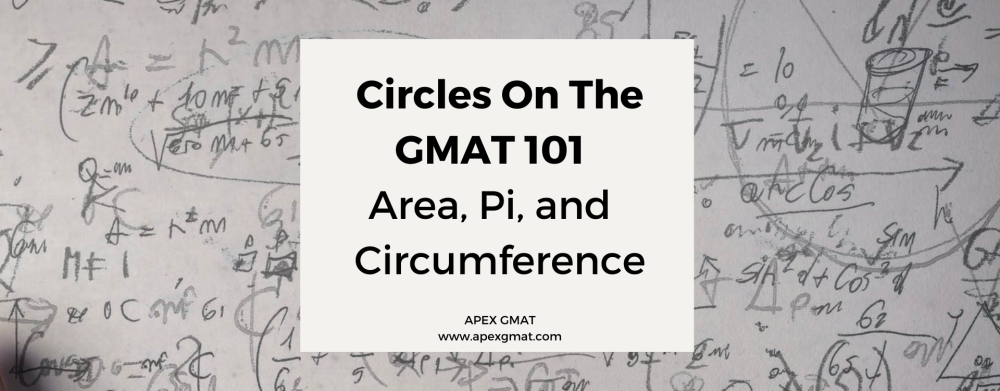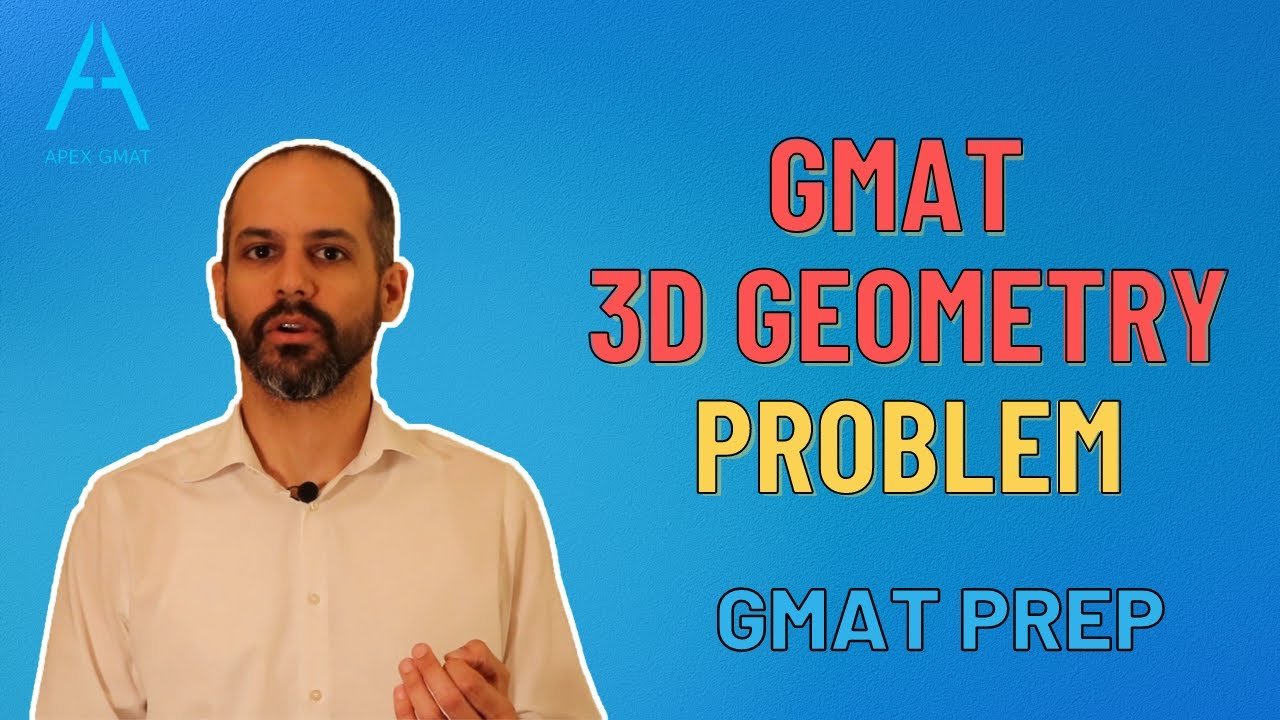## Inscribed Angles & Inscribed Polygons In The GMAT

Welcome back to our third article on GMAT circles. In the second article, we explored central angles, sectors, and arcs. This time we will introduce another kind of angle: the inscribed angle. An inscribed angle is an angle drawn by using line segments to connect one...## Pieces of Pi: Sectors, Arcs, and Central Angles

Welcome back to our series on GMAT circles. In the first article, we introduced the properties of radius/diameter, circumference, and area, discussing the relationships between all of these. This time we will introduce something called a central angle, which creates...## Circles On The GMAT 101 – Area, Circumference, and Pi

Circles on the GMAT function like any other GMAT quant topic: the list of “knowledge bits” you need is short, but the questions creatively combine and/or disguise these few “knowledge bits” to create complex problems.  In this first article, we will discuss the most...## GMAT 3D Geometry Problem – GMAT Math – Quant Section

GMAT 3D Geometry Problem  In this problem we’re going to take a look at 3D objects and in particular a special problem type on the GMAT that measures the longest distance within a three-dimensional object. Typically, they give you rectangular solids, but they...